# Introduction to bvartools

## Introduction

The package bvartools implements functions for Bayesian inference of linear vector autoregressive (VAR) models. It separates a typical BVAR analysis workflow into multiple steps:

• Model set-up: Produces data matrices for given lag orders and model types, which can be used for posterior simulation.
• Prior specification: Generates prior matrices for a given model.
• Estimation: Researchers can choose to use the posterior algorithms of the package or use their own algorithms.
• Standardising model output: Combines the output of the estimation step into standardised objects for subsequent steps of the analyis.
• Evaluation: Produces summary statistics, forecasts, impulse responses and forecast error variance decompositions.

In each step researchers are provided with the opportunitiy to fine-tune a model according to their specific requirements or to use the default framework for commonly used models and priors. Since version 0.1.0 the package comes with posterior simulation functions that do not require to implement any further simulation algorithms. For Bayesian inference of stationary VAR models the package covers

• Standard BVAR models with independent normal-Wishart priors
• BVAR models employing stochastic search variable selection à la Gerorge, Sun and Ni (2008)
• BVAR models employing Bayesian variable selection à la Korobilis (2013)
• Structural BVAR models, where the structural coefficients are estimated from contemporary endogenous variables (A-model)

For Bayesian inference of cointegrated VAR models the package implements the algorithm of Koop, León-González and Strachan (2010) [KLS] – which places identification restrictions on the cointegration space – in the following variants

• The BVEC model as presented in Koop et al. (2010)
• The KLS model employing stochastic search variable selection à la Gerorge, Sun and Ni (2008)
• The KLS modol employing Bayesian variable selection à la Korobilis (2013)
• Structural BVEC models, where the structural coefficients are estimated from contemporaneous endogenous variables (A-model). However, no further restrictions are made regarding the cointegration term.

This introduction to bvartools provides the code to set up and estimate a basic Bayesian VAR (BVAR) model.1 The first part covers a basic workflow, where the standard posterior simulation algorithm of the package is employed for Bayesian inference. The second part presents a workflow for a posterior algorithm as it could be implemented by a researcher.

For both illustrations the data set E1 from Lütkepohl (2006) is used. It contains data on West German fixed investment, disposable income and consumption expenditures in billions of DM from 1960Q1 to 1982Q4. Like in the textbook only the first 73 observations of the log-differenced series are used.

library(bvartools)

data("e1")
e1 <- diff(log(e1)) * 100

# Reduce number of oberservations
e1 <- window(e1, end = c(1978, 4))

# Plot the series
plot(e1)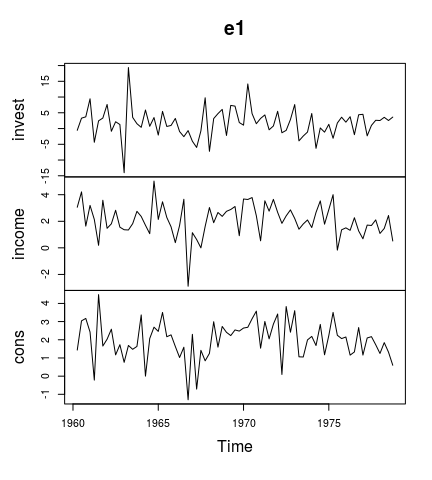## Using bvartools with built-in algorithms

### Setting up a model

The gen_var function produces an object, which contains information on the specification of the VAR model that should be estimated. The following code specifies a VAR(2) model with an intercept term. The number of iterations and burn-in draws is already specified at this stage.

model <- gen_var(e1, p = 2, deterministic = "const",
iterations = 5000, burnin = 1000)

Note that the function is also capable of generating more than one model. For example, specifying p = 0:2 would result in three models.

Function add_priors produces priors for the specified model(s) in object model and augments the object accordingly.

model_with_priors <- add_priors(model,
coef = list(v_i = 0, v_i_det = 0),
sigma = list(df = 1, scale = .0001))

If researchers want to fine-tune individual prior specifications, this can be done by directly accessing the respective elements in object model_with_priors.

### Obtaining posterior draws

Function draw_posterior can be used to produce posterior draws for a model.

bvar_est <- draw_posterior(model_with_priors)
#> Estimating models...

If researchers prefer to use their own posterior algorithms, this can be done by specifying argument FUN with a function that uses obejct model_with_priors as its input. Its output is an object of class bvar (see below).

If multiple models should be estimated, the function allows to make use of parallel computing by specifying argument mc.cores.

### Summary statistics

Summary statistics can be obtained in the usual way using the summary method.

summary(bvar_est)
#>
#> Model:
#>
#> y ~ invest.1 + income.1 + cons.1 + invest.2 + income.2 + cons.2 + const
#>
#> Variable: invest
#>
#>             Mean     SD Naive SD Time-series SD    2.5%     50%    97.5%
#> invest.1 -0.3186 0.1261 0.001783       0.001783 -0.5599 -0.3209 -0.07123
#> income.1  0.1526 0.5506 0.007786       0.007786 -0.9685  0.1531  1.23163
#> cons.1    0.9562 0.6747 0.009542       0.009542 -0.3593  0.9483  2.32125
#> invest.2 -0.1635 0.1268 0.001794       0.001747 -0.4059 -0.1635  0.08210
#> income.2  0.1117 0.5434 0.007685       0.007685 -0.9730  0.1165  1.18389
#> cons.2    0.9385 0.6755 0.009553       0.009553 -0.3934  0.9372  2.28847
#> const    -1.6755 1.7787 0.025154       0.025154 -5.1137 -1.6798  1.77272
#>
#> Variable: income
#>
#>              Mean      SD  Naive SD Time-series SD     2.5%      50%  97.5%
#> invest.1  0.04491 0.03262 0.0004613      0.0004613 -0.01976  0.04460 0.1092
#> income.1 -0.15264 0.14526 0.0020543      0.0020543 -0.44023 -0.15473 0.1336
#> cons.1    0.28777 0.17670 0.0024989      0.0024989 -0.05627  0.28383 0.6387
#> invest.2  0.05039 0.03235 0.0004576      0.0004576 -0.01374  0.05083 0.1141
#> income.2  0.01877 0.13774 0.0019479      0.0019164 -0.25240  0.02034 0.2867
#> cons.2   -0.01024 0.17407 0.0024617      0.0024617 -0.35184 -0.01047 0.3369
#> const     1.57787 0.44980 0.0063611      0.0063611  0.69129  1.57429 2.4567
#>
#> Variable: cons
#>
#>               Mean      SD  Naive SD Time-series SD      2.5%       50%   97.5%
#> invest.1 -0.002211 0.02626 0.0003714      0.0003714 -0.052946 -0.002215 0.04932
#> income.1  0.226626 0.11469 0.0016219      0.0015875 -0.002901  0.227946 0.45138
#> cons.1   -0.264872 0.14128 0.0019979      0.0019979 -0.539186 -0.266096 0.01613
#> invest.2  0.034250 0.02630 0.0003719      0.0003719 -0.016949  0.034341 0.08633
#> income.2  0.354168 0.11270 0.0015939      0.0015336  0.130577  0.352554 0.57702
#> cons.2   -0.022852 0.14009 0.0019812      0.0019812 -0.302435 -0.020934 0.24725
#> const     1.294065 0.36080 0.0051025      0.0051025  0.574392  1.292816 1.98983
#>
#> Variance-covariance matrix:
#>
#>                  Mean     SD Naive SD Time-series SD    2.5%     50%  97.5%
#> invest_invest 22.3342 4.0153 0.056785       0.061974 15.9090 21.8297 31.154
#> invest_income  0.7459 0.7269 0.010280       0.011504 -0.5683  0.7347  2.244
#> invest_cons    1.2961 0.6072 0.008587       0.009441  0.2091  1.2468  2.568
#> income_invest  0.7459 0.7269 0.010280       0.011504 -0.5683  0.7347  2.244
#> income_income  1.4448 0.2669 0.003775       0.004267  1.0258  1.4078  2.053
#> income_cons    0.6476 0.1723 0.002437       0.002687  0.3622  0.6289  1.040
#> cons_invest    1.2961 0.6072 0.008587       0.009441  0.2091  1.2468  2.568
#> cons_income    0.6476 0.1723 0.002437       0.002687  0.3622  0.6289  1.040
#> cons_cons      0.9378 0.1684 0.002382       0.002782  0.6664  0.9192  1.323

As expected for an algrotihm with uninformative priors the posterior means are fairly close to the results of the frequentist estimator, which can be obtaind in the following way:

# Obtain data for LS estimator
y <- t(model$data$Y)
z <- t(model$data$Z)

# Calculate LS estimates
A_freq <- tcrossprod(y, z) %*% solve(tcrossprod(z))

# Round estimates and print
round(A_freq, 3)
#>        invest.1 income.1 cons.1 invest.2 income.2 cons.2  const
#> invest   -0.320    0.146  0.961   -0.161    0.115  0.934 -1.672
#> income    0.044   -0.153  0.289    0.050    0.019 -0.010  1.577
#> cons     -0.002    0.225 -0.264    0.034    0.355 -0.022  1.293

### Thin results

The MCMC series in object est_bvar can be thinned using

bvar_est <- thin_posterior(bvar_est, thin = 10)

### Forecasts

Forecasts with credible bands can be obtained with the function predict. If the model contains deterministic terms, new values can be provided in the argument new_d. If no values are provided, the function sets them to zero. The number of rows of new_d must be the same as the argument n.ahead.

bvar_pred <- predict(bvar_est, n.ahead = 10, new_d = rep(1, 10))

plot(bvar_pred)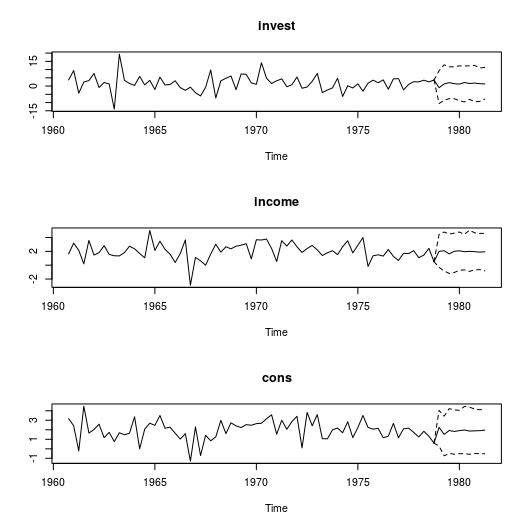### Impulse response analysis

bvartools supports commonly used impulse response functions. See https://www.r-econometrics.com/timeseries/irf/ for an introduction.

#### Forecast error impulse response

FEIR <- irf(bvar_est, impulse = "income", response = "cons", n.ahead = 8)

plot(FEIR, main = "Forecast Error Impulse Response", xlab = "Period", ylab = "Response")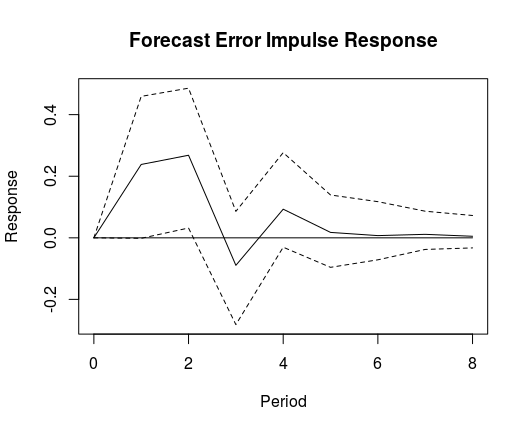#### Orthogonalised impulse response

OIR <- irf(bvar_est, impulse = "income", response = "cons", n.ahead = 8, type = "oir")

plot(OIR, main = "Orthogonalised Impulse Response", xlab = "Period", ylab = "Response")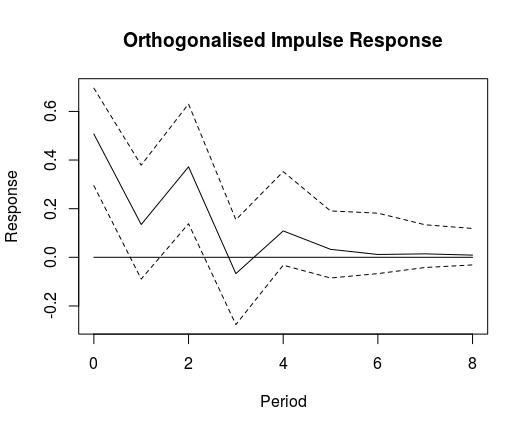#### Generalised impulse response

GIR <- irf(bvar_est, impulse = "income", response = "cons", n.ahead = 8, type = "gir")

plot(GIR, main = "Generalised Impulse Response", xlab = "Period", ylab = "Response")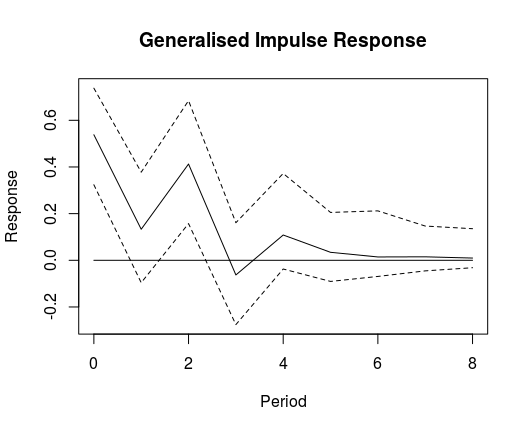### Variance decomposition

bvartools also supports forecast error variance decomposition (FEVD) and generalised forecast error variance decomposition.

#### Forecast error variance decomposition

bvar_fevd_oir <- fevd(bvar_est, response = "cons")

plot(bvar_fevd_oir, main = "OIR-based FEVD of consumption")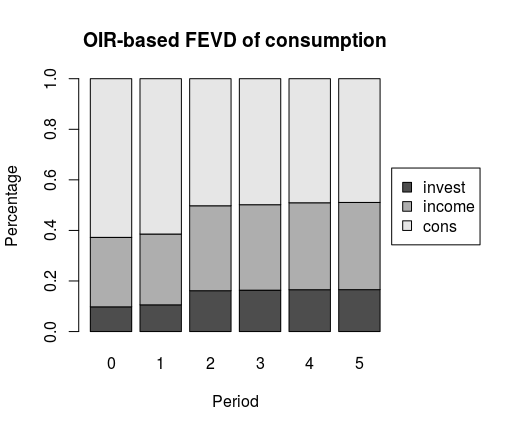#### Generalised forecast error variance decomposition

It is also possible to calculate FEVDs, which are based on generalised impulse responses (GIR). Note that these do not automatically add up to unity. However, this could be changed by adding normalise_gir = TRUE to the function’s arguments.

bvar_fevd_gir <- fevd(bvar_est, response = "cons", type = "gir")

plot(bvar_fevd_gir, main = "GIR-based FEVD of consumption")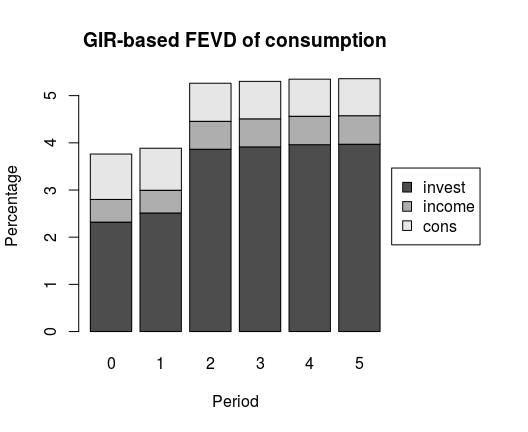## Using bvartools with user-written algorithms

bvartools was created to assist researchers in building and evaluating their own posterior simulation algorithms for linear BVAR models. Functions gen_var and add_priors simply help to quickly obtain the relevant data matrices for posterior simulation. Estimation can be done using algortihms, which are usually implemented by the researchers themselves. But once posterior draws are obtained bvartools can assist throughout in the following steps of the analysis. In this context the main contributions of the package are:

• Functions bvar and bvec collect the output of a Gibbs sampler in standardised objects, which can be used for subsequent steps in an analysis.
• Functions such as predict, irf, fevd for forecasting, impulse response analysis and forecast error variance decomposition, respectively, use the output of bvar and, hence, researchers do not have to implement them themselves and can save time.
• Computationally intensive functions - such as for posterior simulation - are written in C++ using the RcppArmadillo package of Eddelbuettel and Sanderson (2014).2 This decreases calculation time and makes the code less complex and, thus, less prone to mistakes.

If researchers are willing to rely on the model generation and evaluation functions of bvartools, the only remaing step is to illustrate how user specific algorithms can be combined with the functional framework of the package. This is shown in the remainder of this introduction.

### Model set-up and prior specifications

These steps are exactly the same as described above. Thus, the following Gibbs sampler departs from object model_with_priors from above.

### A Gibbs sampler algorithm

# Reset random number generator for reproducibility
set.seed(1234567)

# Get data matrices
y <- t(model_with_priors$data$Y)
x <- t(model_with_priors$data$Z)

tt <- ncol(y) # Number of observations
k <- nrow(y) # Number of endogenous variables
m <- k * nrow(x) # Number of estimated coefficients

# Priors for coefficients
a_mu_prior <- model_with_priors$priors$coefficients$mu # Prior means a_v_i_prior <- model_with_priors$priors$coefficients$v_i # Prior precisions

# Priors for error variance-covariance matrix
u_sigma_df_prior <- model_with_priors$priors$sigma$df # Prior degrees of freedom u_sigma_scale_prior <- model_with_priors$priors$sigma$scale # Prior covariance matrix
u_sigma_df_post <- tt + u_sigma_df_prior # Posterior degrees of freedom

# Initial values for variance-covariance matrix
u_sigma <- diag(.00001, k)
u_sigma_i <- solve(u_sigma)

# Number of iterations of the Gibbs sampler
iterations <- model_with_priors$model$iterations
# Number of burn-in draws
burnin <- model_with_priors$model$burnin
# Total number of draws
draws <- iterations + burnin

# Storate for posterior draws
draws_a <- matrix(NA, m, iterations)
draws_sigma <- matrix(NA, k^2, iterations)

# Start Gibbs sampler
for (draw in 1:draws) {

# Draw conditional mean parameters
a <- post_normal(y, x, u_sigma_i, a_mu_prior, a_v_i_prior)

# Draw variance-covariance matrix
u <- y - matrix(a, k) %*% x # Obtain residuals
u_sigma_scale_post <- solve(u_sigma_scale_prior + tcrossprod(u))
u_sigma_i <- matrix(rWishart(1, u_sigma_df_post, u_sigma_scale_post)[,, 1], k)

# Store draws
if (draw > burnin) {
draws_a[, draw - burnin] <- a
draws_sigma[, draw - burnin] <- solve(u_sigma_i) # Invert Sigma_i to obtain Sigma
}
}

### bvar objects

The bvar function can be used to collect relevant output of the Gibbs sampler into a standardised object, which can be used by functions such as predict to obtain forecasts or irf for impulse respons analysis.

bvar_est_two <- bvar(y = model_with_priors$data$Y,
x = model_with_priors$data$Z,
A = draws_a[1:18,],
C = draws_a[19:21, ],
Sigma = draws_sigma)

Since the output of function draw_posterior is an object of class bvar, the calculation of summary statistics, forecasts, impulse responses and forecast error variance decompositions is performed as described above.

summary(bvar_est_two)
#>
#> Model:
#>
#> y ~ invest.1 + income.1 + cons.1 + invest.2 + income.2 + cons.2 + const
#>
#> Variable: invest
#>
#>             Mean     SD Naive SD Time-series SD    2.5%     50%    97.5%
#> invest.1 -0.3171 0.1287 0.001820       0.001781 -0.5727 -0.3161 -0.06312
#> income.1  0.1465 0.5596 0.007914       0.007914 -0.9499  0.1503  1.24997
#> cons.1    0.9576 0.6908 0.009770       0.009770 -0.3801  0.9519  2.30291
#> invest.2 -0.1599 0.1263 0.001787       0.001787 -0.4050 -0.1609  0.08937
#> income.2  0.1128 0.5458 0.007719       0.007719 -0.9591  0.1133  1.18511
#> cons.2    0.9202 0.6874 0.009721       0.009721 -0.4190  0.9364  2.28972
#> const    -1.6451 1.7712 0.025049       0.025049 -5.1131 -1.6536  1.82543
#>
#> Variable: income
#>
#>              Mean      SD  Naive SD Time-series SD     2.5%      50%  97.5%
#> invest.1  0.04373 0.03269 0.0004623      0.0004623 -0.02191  0.04371 0.1069
#> income.1 -0.15152 0.14307 0.0020233      0.0019753 -0.43908 -0.14968 0.1253
#> cons.1    0.28606 0.17319 0.0024492      0.0024492 -0.04684  0.28727 0.6285
#> invest.2  0.05001 0.03239 0.0004580      0.0004580 -0.01478  0.04986 0.1129
#> income.2  0.02069 0.13831 0.0019559      0.0019559 -0.24879  0.01900 0.2964
#> cons.2   -0.01443 0.17287 0.0024448      0.0024448 -0.34814 -0.01354 0.3203
#> const     1.58537 0.45301 0.0064065      0.0064065  0.70491  1.58560 2.4734
#>
#> Variable: cons
#>
#>               Mean      SD  Naive SD Time-series SD      2.5%       50%
#> invest.1 -0.003013 0.02659 0.0003761      0.0003761 -0.056220 -0.002736
#> income.1  0.223180 0.11491 0.0016250      0.0016250  0.001509  0.224370
#> cons.1   -0.262224 0.13898 0.0019655      0.0020287 -0.538874 -0.257616
#> invest.2  0.034337 0.02588 0.0003660      0.0003660 -0.018436  0.034896
#> income.2  0.355342 0.11217 0.0015863      0.0015863  0.133435  0.357047
#> cons.2   -0.025449 0.13903 0.0019662      0.0019662 -0.297178 -0.025842
#> const     1.297327 0.36338 0.0051389      0.0051389  0.607255  1.287345
#>             97.5%
#> invest.1 0.049540
#> income.1 0.448329
#> cons.1   0.002043
#> invest.2 0.084839
#> income.2 0.572082
#> cons.2   0.254113
#> const    2.013921
#>
#> Variance-covariance matrix:
#>
#>                  Mean     SD Naive SD Time-series SD    2.5%     50%  97.5%
#> invest_invest 22.3994 4.0777 0.057668       0.065232 15.9104 21.8679 31.819
#> invest_income  0.7388 0.7260 0.010267       0.011516 -0.6131  0.7156  2.268
#> invest_cons    1.2950 0.5977 0.008452       0.009363  0.2147  1.2703  2.534
#> income_invest  0.7388 0.7260 0.010267       0.011516 -0.6131  0.7156  2.268
#> income_income  1.4395 0.2677 0.003786       0.004205  1.0158  1.4042  2.057
#> income_cons    0.6422 0.1690 0.002391       0.002679  0.3537  0.6262  1.017
#> cons_invest    1.2950 0.5977 0.008452       0.009363  0.2147  1.2703  2.534
#> cons_income    0.6422 0.1690 0.002391       0.002679  0.3537  0.6262  1.017
#> cons_cons      0.9352 0.1678 0.002373       0.002649  0.6597  0.9183  1.318

1. Further examples about the use of the bvartools package are available at https://www.r-econometrics.com/timeseriesintro/.

2. RcppArmadillo is the Rcpp bridge to the open source ‘Armadillo’ library of Sanderson and Curtin (2016).